Math Olympiad Test: Arithmetic Progression- 2

# Math Olympiad Test: Arithmetic Progression- 2 - Class 10

Test Description

## 15 Questions MCQ Test Olympiad Preparation for Class 10 - Math Olympiad Test: Arithmetic Progression- 2

Math Olympiad Test: Arithmetic Progression- 2 for Class 10 2023 is part of Olympiad Preparation for Class 10 preparation. The Math Olympiad Test: Arithmetic Progression- 2 questions and answers have been prepared according to the Class 10 exam syllabus.The Math Olympiad Test: Arithmetic Progression- 2 MCQs are made for Class 10 2023 Exam. Find important definitions, questions, notes, meanings, examples, exercises, MCQs and online tests for Math Olympiad Test: Arithmetic Progression- 2 below.
 1 Crore+ students have signed up on EduRev. Have you?
Math Olympiad Test: Arithmetic Progression- 2 - Question 1

### Find the sum of all two-digits odd positive integers.

Detailed Solution for Math Olympiad Test: Arithmetic Progression- 2 - Question 1

Given AP is 11 + 13 + 15 + …..+ 99
Here a = 11, d = 13 - 11 = 2, l = 99
l = a + (n - 1)d
⇒ 99 = 11 + (n - 1)2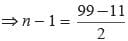⇒ n - 1 = 44
⇒ n = 45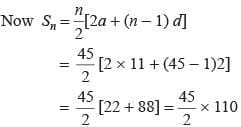= 45 × 55 = 2475

Math Olympiad Test: Arithmetic Progression- 2 - Question 2

### What is the nth term of the sequence log a, log ab, log ab2, log ab3, . . .

Detailed Solution for Math Olympiad Test: Arithmetic Progression- 2 - Question 2

loga, logab, logab2, logab3, ….
loga, loga + logb, loga + 2logb, loga + 3logb
t1 = loga, d = loga + logb - loga = logb
tn = t1 + (n - 1)d = loga + (n - 1)logb
= loga + logbn-1
= logabn-1

Math Olympiad Test: Arithmetic Progression- 2 - Question 3

### If the sum of n terms of an arithmetic progression is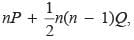where P and Q are constants. What is the common difference?

Detailed Solution for Math Olympiad Test: Arithmetic Progression- 2 - Question 3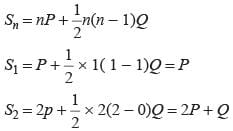S2 - S1 = 2P + Q - P = P + Q
P, P + Q ….
Common difference = Q

Math Olympiad Test: Arithmetic Progression- 2 - Question 4

The sum of n terms of the three arithmetic progression are S1, S2, S3. The first term of each arithmetic progression is unity. The common differences are 1, 2, 3 respectively, then which of the following options is correct?

Detailed Solution for Math Olympiad Test: Arithmetic Progression- 2 - Question 4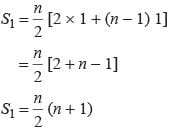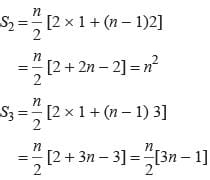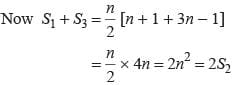Math Olympiad Test: Arithmetic Progression- 2 - Question 5

The sum of three numbers in arithmetic is 27 and their product is 405. What are the numbers?

Detailed Solution for Math Olympiad Test: Arithmetic Progression- 2 - Question 5

Let the numbers be a - d, a, a + d
Now a - d + a + a +d = 27 ⇒ a = 9
and (a - d) a (a + d) = 405
a(a2 - d2) = 405
9(92 - d2) = 405
⇒ 81 - a2 = 45
⇒ d2 = 36 ⇒ d = ±6
The numbers are 3, 9, 15.

Math Olympiad Test: Arithmetic Progression- 2 - Question 6

If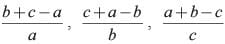are in arithmetic progression then which of the following is correct?

Detailed Solution for Math Olympiad Test: Arithmetic Progression- 2 - Question 6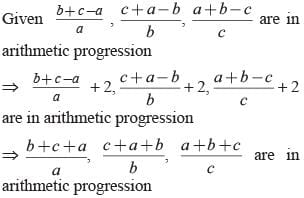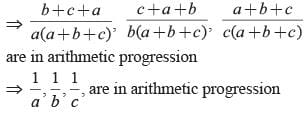Math Olympiad Test: Arithmetic Progression- 2 - Question 7

What is the sum of all three digit natural numbers which are multiples of 7?

Detailed Solution for Math Olympiad Test: Arithmetic Progression- 2 - Question 7

The three-digit natural numbers which are multiples of 7 are
105, 112, 119,…, 994
a2 – a1 = 112 – 105 = 7
a3 – a2 = 112 – 105 = 7
∵ a3 – a2 = a2 – a1 = 7
Therefore, the series is in AP
Here, a = 105, d = 7 and an = 994
We know that,
an = a + (n – 1)d
⇒ 994 = 105 + (n – 1)7
⇒ 994 – 105 = (n – 1)7
⇒ 889 = (n – 1)7
⇒ 127 = (n – 1)
⇒ n = 128
Now, we have to find the sum of this AP⇒ S128 = 64[210 + 127 × 7]
⇒ S128 = 64
⇒ S128 = 70336
Hence, the sum of all three-digit numbers which are multiples of 7 is 70336.

Math Olympiad Test: Arithmetic Progression- 2 - Question 8

The sum of first seven terms of an arithmetic progression is 10 and the sum of next seven terms is 17, then what is the common difference of this arithmetic progression?

Detailed Solution for Math Olympiad Test: Arithmetic Progression- 2 - Question 8

Given: Sum of first 7 terms, S7 = 10
and Sum of the next 7 terms = 17
⇒ Sum of 8th to 14th terms = 17
⇒ Sum of first 14 terms – Sum of first 7 terms = 17
⇒ S14 – S7 = 17
⇒ S14 – 10 = 17
⇒ S14 = 27

Math Olympiad Test: Arithmetic Progression- 2 - Question 9

If in an arithmetic progression the sum of m terms is n and the sum of n terms is m then what is the sum of (m + n) terms?

Detailed Solution for Math Olympiad Test: Arithmetic Progression- 2 - Question 9

Let a be the first term and d be the common difference.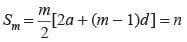2am + m(m - 1)d = 2n (1)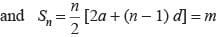2an + n(n - 1)d = 2m (2)
Subtracting (2) from eqn (1), we get
2a(m - n) + [(m2 - n2) - (m - n)]d = 2(n - m)
⇒ (m - n)[2a + (m + n - 1) d] = 2(n - m)
2a + (m + n - 1)d = -2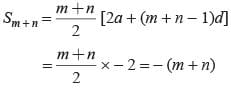Math Olympiad Test: Arithmetic Progression- 2 - Question 10

If pth, qth and rth terms of an arithmetic progression are a, b, c respectively, then what is the value of a(q - r) + b(r - p) + c(p - q)?

Detailed Solution for Math Olympiad Test: Arithmetic Progression- 2 - Question 10

Let x be the first term and d be the common difference.
pth term = a
x + (p - 1)d = a ...(1)
qth term = b ⇒ x + (q - 1)d = b ...(2)
rth term = c ⇒ x + (r - 1)d = c ...(3)
∴ a(q - r) + b(r - p) + c(p - q)
= x[q - r + r - p + p - q] + d[(p - 1) (q - r) + (q - 1) (r - p) + (r - 1) (q - q)]
⇒ a(q - r) + b(r - p) + c(p - q) = 0

Math Olympiad Test: Arithmetic Progression- 2 - Question 11

Find the sum of n terms of an arithmetic progression whose kth term is 5k + 1

Detailed Solution for Math Olympiad Test: Arithmetic Progression- 2 - Question 11

tk = 5k + 1
t1 = 5 × 1 + 1 = 6
tn = 5n + 1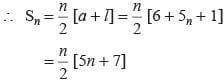Math Olympiad Test: Arithmetic Progression- 2 - Question 12

How many terms of an arithmetic progression 3, 7, 11, 15, …. are taken so that the sum is 406?

Detailed Solution for Math Olympiad Test: Arithmetic Progression- 2 - Question 12

3, 7, 11, 15…..
a = 3, d = 4, Sn = 406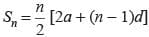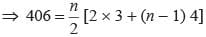⇒ n[6 + 4n - 4] = 812
⇒ 4n2 + 2n = 812
⇒ 2n2 + n = 406
⇒ 2n2 + 29n - 28n - 406 = 0
⇒ n(2n + 29) × 14(2n + 29) = 0
⇒ (n - 14) (2n + 29) = 0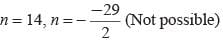Math Olympiad Test: Arithmetic Progression- 2 - Question 13

What is the value of k for which 5k + 2, 4k - 1 and k + 2 are in arithmetic progression?

Detailed Solution for Math Olympiad Test: Arithmetic Progression- 2 - Question 13

Here 4k - 1 - (5k + 2) = (k + 2) - (4k - 1)
⇒ 4k - 1 - 5k - 2 = k + 2 - 4k + 1
⇒ -k - 3 = -3k + 3
⇒ 2k = 6 ⇒ k = 3

Math Olympiad Test: Arithmetic Progression- 2 - Question 14

If the mth term of an arithmetic is 1/n and its nth term 1/m then what is its (mn)th term?

Detailed Solution for Math Olympiad Test: Arithmetic Progression- 2 - Question 14

Let a be the first term and d be the common difference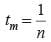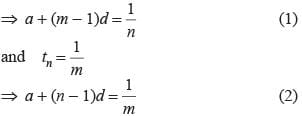Subtracting eqn (2) from eqn (1)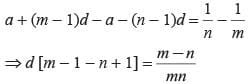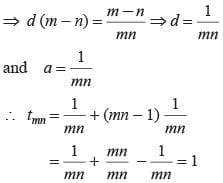Math Olympiad Test: Arithmetic Progression- 2 - Question 15

The first, second and last term of an arithmetic progression are respectively 4, 7 and 31. How many terms are there in the given arithmetic progression?

Detailed Solution for Math Olympiad Test: Arithmetic Progression- 2 - Question 15

a = 4, d = 7 - 4 = 3, tn = 31
∴ tn = a + (n - 1)d
⇒ 31 = 4 + (n - 1) 3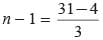⇒ n - 1 = 9 ⇒ n = 10

## Olympiad Preparation for Class 10

11 videos|36 docs|201 tests